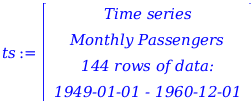Units - Maple Help

UnitsIntroduction Maple 2018 offers enhanced tools for using units throughout your analysis.Units in Solving, Integration, and Optimization

You can now use use units in numeric equation solving, integration, and optimization. Several functions support units, including fsolve and the optimizers in the Optimization package. In addition, units handling has been improved in the solve command and the int command for symbolic and numeric inputs.Example: Adiabatic Flame Temperature of Butane

Liquid butane is burnt with 100% theoretical air at an initial temperature of 298.15 K. The combustion reaction is

C4H10 (l) + 6.5 O2 (g)+ 24.44 N2 (g) → 4 CO2 (g) + 5 H2O (g) + 24.44 N2 (g)

Here, we will calculate the adiabatic flame temperature of the combustion products.

 > $\mathrm{with}\left(\mathrm{ThermophysicalData}\right):$

Heat of formation of butane

 > $\mathrm{h_f_C4H10}≔\mathrm{Chemicals}:-\mathrm{Property}\left("HeatOfFormation","C4H10\left(l\right),n-buta",\mathrm{useunits}\right)$
 ${-}{150.6640000}{}⟦\frac{{\mathrm{kJ}}}{{\mathrm{mol}}}⟧$ (2.1.1)

Enthalpies of the combustion products at a temperature T

 > $\mathrm{h_N2}≔\mathrm{Chemicals}:-\mathrm{Property}\left("Hmolar","N2\left(g\right)","temperature"=\mathrm{T}\right):$$\phantom{\rule[-0.0ex]{0.0em}{0.0ex}}\mathrm{h_O2}≔\mathrm{Chemicals}:-\mathrm{Property}\left("Hmolar","O2\left(g\right)","temperature"=\mathrm{T}\right):\phantom{\rule[-0.0ex]{0.0em}{0.0ex}}\phantom{\rule[-0.0ex]{0.0em}{0.0ex}}$$\mathrm{h_H2O}≔\mathrm{Chemicals}:-\mathrm{Property}\left("Hmolar","H2O\left(g\right)","temperature"=\mathrm{T}\right):\phantom{\rule[-0.0ex]{0.0em}{0.0ex}}\phantom{\rule[-0.0ex]{0.0em}{0.0ex}}$$\mathrm{h_CO2}≔\mathrm{Chemicals}:-\mathrm{Property}\left("Hmolar","CO2\left(g\right)","temperature"=\mathrm{T}\right):$

Enthalpy of the reactants

 >
 ${-}{150.6640000}{}⟦{\mathrm{kJ}}⟧$ (2.1.2)

Total enthalpy of the combustion products

 >
 ${\mathrm{H_products}}{≔}{4}{}{\mathrm{Chemicals}}{:-}{\mathrm{Property}}{}\left({"Hmolar"}{,}{"CO2\left(g\right)"}{,}{"temperature"}{=}{T}\right){}⟦{\mathrm{mol}}⟧{+}{5}{}{\mathrm{Chemicals}}{:-}{\mathrm{Property}}{}\left({"Hmolar"}{,}{"H2O\left(g\right)"}{,}{"temperature"}{=}{T}\right){}⟦{\mathrm{mol}}⟧{+}{24.44}{}{\mathrm{Chemicals}}{:-}{\mathrm{Property}}{}\left({"Hmolar"}{,}{"N2\left(g\right)"}{,}{"temperature"}{=}{T}\right){}⟦{\mathrm{mol}}⟧$ (2.1.3)

Equating the enthalpy of the reactants and the enthalpy of the combustion products gives the adiabatic flame temperature

 > $\mathrm{fsolve}\left(\mathrm{H_reactants}=\mathrm{H_products},\mathrm{T}=2000⟦\mathrm{K}⟧\right)$
 ${2379.853026}{}⟦{K}⟧$ (2.1.4)Example: Optimizing the Design of a Fuel Pod

You are designing a fuel pod with a hemispherical cap, cylindrical mid-section and conical cap.What are values of L, H and R that minimize the surface area while maintaining the volume V at 3 m3?

 > $\mathrm{restart}:$

Objective function - surface area of pod

 > $\mathrm{obj}≔\frac{1}{2}\cdot 4\cdot \mathrm{\pi }\cdot {\mathrm{R}}^{2}+2\cdot \mathrm{\pi }\cdot \mathrm{R}\cdot \mathrm{L}+\mathrm{\pi }\cdot \mathrm{R}\cdot \sqrt{{\mathrm{H}}^{2}+{\mathrm{R}}^{2}}:$

Constraint on the volume area of pod

 >

All dimensions must be greater than 0

 > $\mathrm{cons2}≔0\le \mathrm{R},0\le \mathrm{L},0\le \mathrm{H}:$

Hence the optimized dimensions are

 > $\mathrm{dimensions}≔\mathrm{Optimization}:-\mathrm{Minimize}\left(\mathrm{obj},\left\{\mathrm{cons1},\mathrm{cons2}\right\},\mathrm{initialpoint}=\left\{\mathrm{H}=1⟦\mathrm{m}⟧,\mathrm{L}=1⟦\mathrm{m}⟧,\mathrm{R}=1⟦\mathrm{m}⟧\right\}\right)$
 $\left[{10.2533536615869920}{}⟦{{m}}^{{2}}⟧{,}\left[{H}{=}{0.785093823049978}{}⟦{m}⟧{,}{L}{=}{0.392546902492684}{}⟦{m}⟧{,}{R}{=}{0.877761593519080}{}⟦{m}⟧\right]\right]$ (2.2.1)Example: Work Done in Isothermal Compression of Methane

Methane at 350 K is isothermally compressed from a specific volume of 1.0 m3 kg-1 to 0.5 m3 kg-1. Here, we will calculate the work done.

First, we define an expression that gives the pressure of methane as a function of the specific volume V.

 > $P≔\mathrm{ThermophysicalData}:-\mathrm{Property}\left("pressure","methane","temperature"=350⟦K⟧,"density"=\frac{1}{V}\right):$

We then perform the numeric integration  to calculate the work done.

 >
 ${125.4345869}{}⟦\frac{{\mathrm{kJ}}}{{\mathrm{kg}}}⟧$ (2.3.1)Unit Conversion

Maple 2018 moves the units formatting dialog into the new Context Panel, and enhances its functionality.Changing units takes fewer mouse clicks, and can be used in tandem with numeric formatting to quickly create presentable, readable results. For example, you can reformat this result...

 > $\mathrm{with}\left(\mathrm{ThermophysicalData}:-\mathrm{Chemicals}\right):\phantom{\rule[-0.0ex]{0.0em}{0.0ex}}\phantom{\rule[-0.0ex]{0.0em}{0.0ex}}\mathrm{Property}\left("Hmolar","C2H5OH\left(L\right)","temperature"=320⟦\mathrm{K}⟧\right)$
 ${-}{274957.7153}{}⟦\frac{{J}}{{\mathrm{mol}}}⟧$ (3.1)

...to...

 > $\mathrm{Property}\left("Hmolar","C2H5OH\left(L\right)","temperature"=320⟦\mathrm{K}⟧\right)$
 ${-}{274.9577153}{}⟦\frac{{\mathrm{kJ}}}{{\mathrm{mol}}}⟧$ (3.2)
 >

...with a few mouse clicks, and without navigating between different dialogs.

The list of suggested unit conversions now gives you

 • typeset Greek letters, e.g. micro Joules are typeset as μJ
 • typeset math, e.g.  instead of J/mol/K
 • sensible suggestions for unit conversions, e.g. selecting a result with units of  offers $\frac{\mathrm{J}}{\mathrm{mol}}$ and $\frac{\mathrm{kJ}}{\mathrm{mol}}$ as potential conversions
 • a cleaner, more streamlined experience by removing little used unit systems (although all unit systems can still be set via the command line)

 Applications